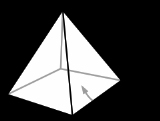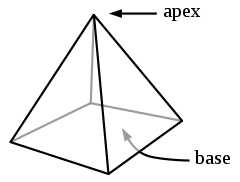xApex (geometry)EncyclopediaIn geometry
Geometry
Geometry arose as the field of knowledge dealing with spatial relationships. Geometry was one of the two fields of pre-modern mathematics, the other being the study of numbers ....

, an apex (Latin
Latin
Latin is an Italic language originally spoken in Latium and Ancient Rome. It, along with most European languages, is a descendant of the ancient Proto-Indo-European language. Although it is considered a dead language, a number of scholars and members of the Christian clergy speak it fluently, and...

for 'summit') is the vertex
Vertex (geometry)
In geometry, a vertex is a special kind of point that describes the corners or intersections of geometric shapes.-Of an angle:...

which is in some sense the highest of the figure to which it belongs.
• In an isosceles triangle
Triangle
A triangle is one of the basic shapes of geometry: a polygon with three corners or vertices and three sides or edges which are line segments. A triangle with vertices A, B, and C is denoted ....

, the apex is the vertex where the two sides of equal length meet, opposite the unequal third side.
• In a pyramid
Pyramid (geometry)
In geometry, a pyramid is a polyhedron formed by connecting a polygonal base and a point, called the apex. Each base edge and apex form a triangle. It is a conic solid with polygonal base....

or cone
Cone (geometry)
A cone is an n-dimensional geometric shape that tapers smoothly from a base to a point called the apex or vertex. Formally, it is the solid figure formed by the locus of all straight line segments that join the apex to the base...

, the apex is the vertex opposite the base
Base (geometry)
In geometry, a base is a side of a plane figure or face of solid, particularly one perpendicular to the direction height is measured or on what is considered to the bottom. This usage can be applied to a triangle, parallelogram, trapezoids, cylinder, pyramid, parallelopiped or frustum. By...

.